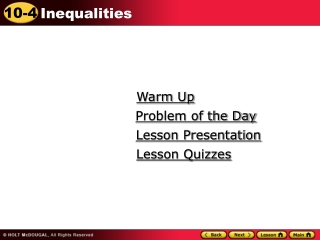DownloadDownload PresentationWarm Up

# Warm Up

Télécharger la présentation## Warm Up

- - - - - - - - - - - - - - - - - - - - - - - - - - - E N D - - - - - - - - - - - - - - - - - - - - - - - - - - -
##### Presentation Transcript

1. Warm Up Problem of the Day Lesson Presentation Lesson Quizzes

2. Warm Up Solve 1.-21z + 12 =-27z 2.-12n - 18 = -6n 3.12y - 56 = 8y 4. -36k + 9 = -18k z = -2 n = -3 y = 14 k = 1 2

3. Problem of the Day The dimensions of one rectangle are twice as large as the dimensions of another rectangle. The difference in the area is 42 cm. What is the area of each rectangle? 56 cm2 and 14 cm2

4. Vocabulary inequality algebraic inequality solution set compound inequality

5. An inequality states that two quantities either are not equal or may not be equal. An inequality uses one of the following symbols:

6. An inequality that contains a variable is an algebraic inequality . A value of the variable that makes the inequality true is a solution of the inequality. An inequality may have more than one solution. Together, all of the solutions are called the solution set. A compound inequality is the result of combining two inequalities. The words and and or are used to describe how the two parts are related.

7. Additional Example 1: Writing Inequalities Write an inequality for each situation. A. There are at least 15 people in the waiting room. number of people ≥ 15 B. The tram attendant will allow no more than 60 people on the tram. number of people ≤ 60

8. Check It Out Example 1 A. There should be no more than 10 people on an elevator number of people ≤ 10 B. There must be at least 20 people signed up for a class or it will be canceled. number of people ≥ 20

9. Additional Example 2: Graphing Simple Inequalities Graph each inequality. n<3. a>=24

10. Check It Out Example 2 Graph each inequality. n<-1 j<=0

11. Additional Example 3A : Graphing Compound Inequalities Graph each compound inequality. m ≤ –2 or m > 1 Graph m ≤ –2. Graph m > 1.

12. Continued: Example 3A Graph the common solutions.

13. Additional Example 3B : Graphing Compound Inequalities -3 < b ≤ 0 Graph -3 < b. Graph b ≤ 0

14. Continued: Example 3B Graph the common solutions.

15. Check It Out Example 3 Graph each compound inequality. Graph x > 4 Graph x < 5.

16. Continued: Check It Out Example 3 Graph the common solutions.

17. Lesson Quizzes Standard Lesson Quiz Lesson Quiz for Student Response Systems

18. Lesson Quiz Write an inequality for each situation 1. No more than 220 people are in the theater. people in the theater ≤ 220 2. There are at least a dozen eggs left. number of eggs ≥ 12 3. Fewer than 14 people attended the meeting. people attending the meeting <14

19. Continued: Lesson Quiz Graph the inequality 4.x>-1. Graph the compound inequality 5.x ≥ -4 or x<-1

20. Lesson Quiz for Student Response Systems Write an inequality for the situation. The bag should hold no more than 4 pounds number of pounds ≥ 4 B. number of pounds ≤ 4 C. number of pounds > 4 D. number of pounds < 4

21. Continued: Lesson Quiz for Student Response Systems 2. Write the inequality. A. x > 1 B. x< 1 C. x ≥ 1 D.x ≤ 1

22. Continued: Lesson Quiz for Student Response Systems 3. Write the inequality. A. –2≤ x ≤ 5 B. –2≥ x ≥ 5 C. –2> x ≤ 5 D. –2≤ x < 5